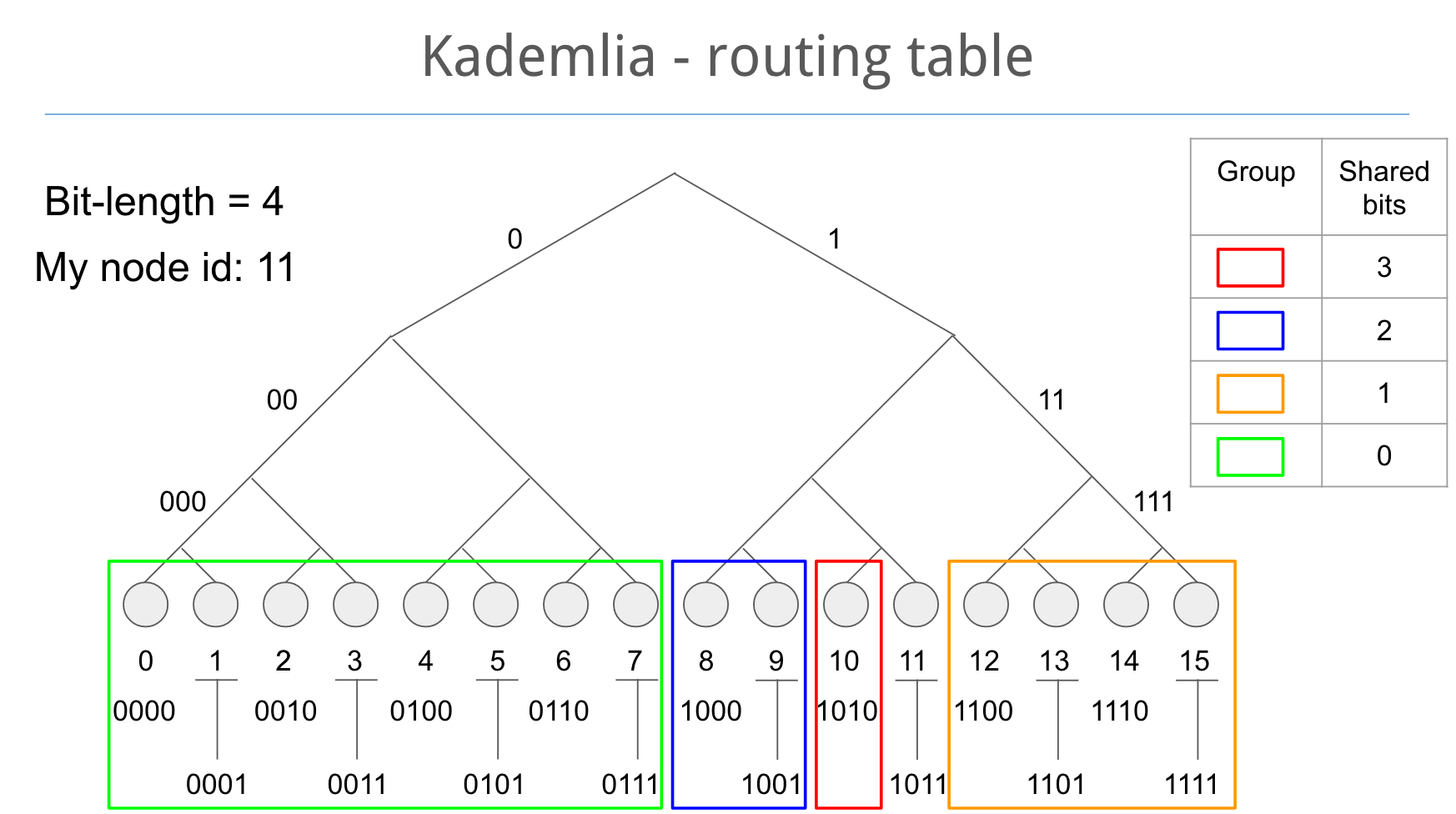Kademlia协议是一种分布式散列表(Distributed Hash Table, DHT) 技术。它可以被用来构建 P2P 网络，相比其他的散列表技术，提高了路由的查询速度。

• $A \bigotimes B = B \bigotimes A$：A 到 B 和 B 到 A 的距离是一样的
• $A \bigotimes A = 0$：节点自身和自身的距离是0
• $A \bigotimes B > 0$：任意两个不同节点之间的距离大于0
• $(A \bigotimes B) + (B \bigotimes C) \ge (A \bigotimes C)$：A 经过 B 到达 C 的距离大于 A 直接到达 C 的距离

## K-bucket(待续，抽空写)